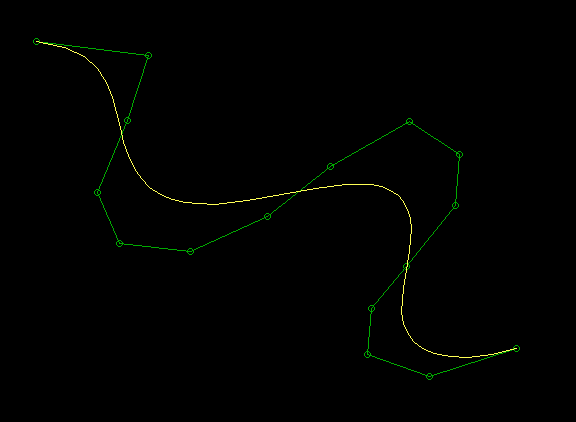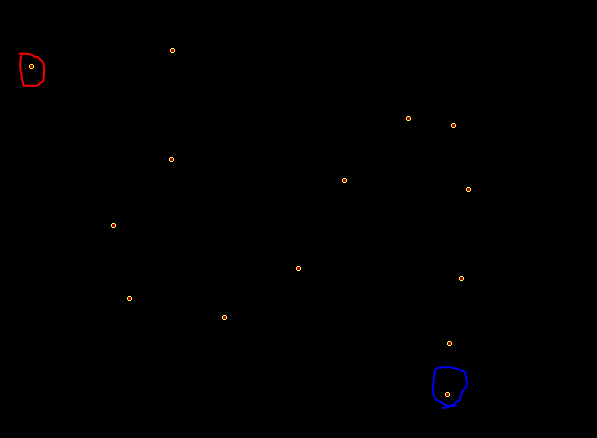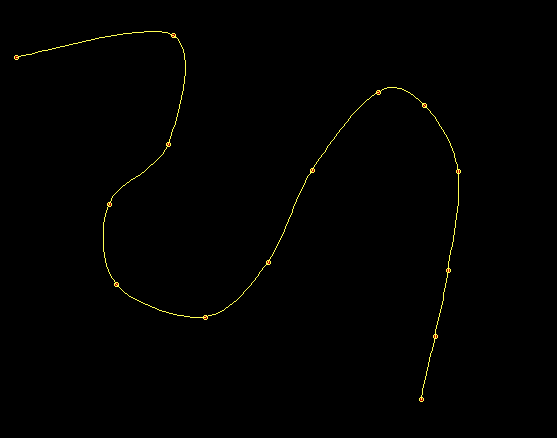• 2021-05-21 06:41:21

该楼层疑似违规已被系统折叠 隐藏此楼查看此楼

我这种方法为什么图形只能显示一下就不见了，这是为什么？能不能用在双曲线上？？能的话改哪里？？

printf("\n 1.input coefficient");

printf("\n 2.input extent");

printf("\n 3.draw function curve");

printf("\n 4.quit");

printf("\n*****************\n");}

void fun1()

{ printf("input a,b,c\n");

scanf("%f,%f,%f",&a,&b,&c);

}void fun2()

{ printf("input xmin,xmax\n"); scanf("%f,%f",&xmin,&xmax);}

void funmax()

{ float m,n; if(xmin<0) m=-xmin; else m=xmin; if(xmax<0) n=-xmax; else n=xmax; if(m>=n) max=m; else max=n;}

float funx(float max_x)

{ return(max_x/2/max);}float funy(float max_y)

{float d; d=max_y/2/(a*max*max+b*max+c); if(d>0.6) d=0.6; if(d<0.0026) d=0.0026; return(d);}

void fun3()

{ int max_x,max_y; int graphdriver=DETECT,graphmode;

float x1,y1,x2,y2,x,coe_x,coe_y; initgraph(&graphdriver,&graphmode,"");

cleardevice(); max_x=getmaxx(); max_y=getmaxy();

funmax();

coe_x=funx(max_x); coe_y=funy(max_y); line(0,240,640,240); line(320,0,320,480); line(640,240,635,235); line(640,240,635,245); line(320,0,315,5); line(320,0,325,5); outtextxy(320,240,"(0,0)");

outtextxy(620,240,"x"); outtextxy(320,10,"y");

x1=max_x/2+xmin*coe_x,y1=max_y/2-(a*xmin*coe_x*xmin*coe_x+b*xmin*coe_x+c)*coe_y;

moveto((int)x1,(int)y1); for(x=xmin*coe_x;x<=xmax*coe_x;x++)

{ x2=max_x/2+x,y2=max_y/2-(a*x*x+b*x+c)*coe_y;

lineto((int)x2,(int)y2); }void getch();closegraph(); }

void main()

{ scanf("%d",&n); switch(n)

{ case 1:fun1();break;

case 2:fun2();break;

case 3:fun3();break;

case 4:exit(0);

default:printf("\n error \n"); } }}

更多相关内容
• 本压缩包里有用Vc++缩写的用迭代法计算信道容量，并画出信道容量随着某一个参数变化时的曲线。开发要用openGL，里面有关于它的一些例子，学起来很容易。自己可以用它画出任意图形的。
• 本文实例为大家分享了C++绘制正弦曲线的具体代码，供大家参考，具体内容如下 通过OnDraw函数绘制图形 过程为先绘制两个坐标轴，再绘制正弦曲线的方法。其中将曲线分解为660个像素点，让其看起来更像“曲线” void...
• C++实现的三次贝塞尔曲线绘制，可拖动控制点修改曲线形状，包含源代码和可执行文件，VS2008的工程。 C++实现的三次贝塞尔曲线绘制，可拖动控制点修改曲线形状，包含源代码和可执行文件，VS2008的工程。
• - 余弦函数 - 正弦函数 #include<iostream> #include<cmath> #define PI 3.1 #define tow_PI 6.2 using namespace std; int main() { double y,x; for(y=1;y>0;y-=0.1) { double cx=asin(y);...

- 余弦函数- 正弦函数

#include<iostream>
#include<cmath>
#define PI 3.1
#define tow_PI 6.2
using namespace std;
int main()
{
double y,x;
for(y=1;y>0;y-=0.1)
{
double cx=asin(y);
for(x=0;x<cx;x+=0.1) cout<<" ";
cout<<"*";
for(;x<PI-cx;x+=0.1) cout<<" ";
cout<<"*"<<endl;
}
for(;y>=-1;y-=0.1)
{
for(double t=0;t<PI;t+=0.1) cout<<" ";
double cx=abs(asin(y));
for(x=0;x<cx;x+=0.1) cout<<" ";
cout<<"*";
for(;x<PI-cx;x+=0.1) cout<<" ";
cout<<"*"<<endl;
}
return 0;
}余弦函数实现简单，只要绘制出0~pi的曲线，然后进行一个对称的绘制就行，因为余弦函数关于x=pi对称。
正弦实现分为两部，上半部分曲线和下半部分曲线。
（ps:反余弦函数和反正弦函数定义域都是-pi~pi,故当绘制正弦函数下半部分曲线时要取绝对值）

展开全文• 绘制Bezier曲线 // 功 能：单击鼠标左键进行折点确定，单击右键进行Bezier曲线（绿色）绘制，同时也会画出多边折线（黄色） // 编译环境：Visual C++ 6.0，EasyX_20190219(beta) #include<graphics.h> #...

绘制Bezier曲线

// 功    能：单击鼠标左键进行折点确定，单击右键进行Bezier曲线（绿色）绘制，同时也会画出多边折线（黄色）
// 编译环境：Visual C++ 6.0，EasyX_20190219(beta)
#include<graphics.h>
#include<conio.h>
double get(int i,int n,double t){
int j;
double a=1,b=1,c=1,d=1,e=1;
for(j=1;j<=n;j++){
a=a*j;
if(j<=i){
b=b*j;
d=d*t;
}
if(j<=n-i){
c=c*j;
e=e*(1.0-t);
}
}
if(a==0){a=1;}
if(b==0){b=1;}
if(c==0){c=1;}
return (a/(b*c))*d*e;
}
void paint_bezier(POINT *p,int n){
int i,j;
double b;
double t=0,x=0,y=0;
setcolor(YELLOW);
moveto(p.x,p.y);
int k=50;
for(i=0;i<k;i++){
t=(i)/double(k);
x=0;y=0;
for(j=0;j<n;j++){
b[j]=get(j,n-1,t);
x=x+b[j]*p[j].x;
y=y+b[j]*p[j].y;
}
lineto(int(x),int(y));
}
lineto(p[n-1].x,p[n-1].y);
}
void paint_outside(POINT *p,int n){
int i;
setcolor(GREEN);
moveto(p.x,p.y);
for(i=0;i<n;i++){
lineto(p[i].x,p[i].y);
}
}
void main(){
int n=0;
initgraph(640,480);
MOUSEMSG msg;
POINT p;
while (true){
msg = GetMouseMsg();
if (msg.uMsg == WM_LBUTTONDOWN){//初始点击鼠标左键绘制坐标点
setcolor(GREEN);
circle(msg.x, msg.y, 3);
p[n].x=msg.x;
p[n].y=msg.y;
n++;
}
if (msg.uMsg == WM_RBUTTONDOWN){//点击鼠标右键退出循环，绘制抛物线
paint_outside(p,n);
paint_bezier(p,n);
n=0;
}
}
_getch();
closegraph();
}


演示如下：绘制抛物样条曲线

//功    能：鼠标控制绘制抛物样条曲线，左键单击时绘制实心圆，右键单击后绘制抛物线
// 编译环境：Visual C++ 6.0，EasyX_20190219(beta)
#include<iostream>
#include<graphics.h>
#include<conio.h>
#include<stdio.h>
#include<math.h>
using namespace std;
void parspl(int p[],int n,int k,int e){
int x,y,i,j,m;
double t1,t2,t3,t,a,b,c,d;
if(e==1){
m=n;
p=p;
p=p;
p[n+1]=p[n];
p[n+1]=p[n];
}
else{
m=n+1;
p=p[n];
p=p[n];
p[m]=p;
p[m]=p;
p[m+1]=p;
p[m+1]=p;
}
t=0.5/k;
moveto(p,p);
for(i=0;i<m-1;i++){
for(j=1;j<k;j++){
t1=j*t;t2=t1*t1;t3=t2*t1;
a=-4.0*t3+4.0*t2-t1;
b=12.0*t3-10.0*t2+1.0;
c=-12.0*t3+8.0*t2+t1;
d=4.0*t3-2.0*t2;
x=a*p[i]+b*p[i+1]+c*p[i+2]+d*p[i+3];
y=a*p[i]+b*p[i+1]+c*p[i+2]+d*p[i+3];
lineto(x,y);
}
lineto(p[i+2],p[i+2]);
}
}

int main()
{
int n = 0, e, k = 15;
e = 1;
initgraph(640, 480);
MOUSEMSG msg;
int p;
setcolor(YELLOW);
setfillcolor(RED);
while (true){
msg = GetMouseMsg();
if (msg.uMsg == WM_LBUTTONDOWN) //初始点击鼠标左键绘制坐标点
{
fillcircle(msg.x, msg.y, 2);
p[n + 1] = msg.x;
p[n + 1] = msg.y;
n++;
}
if (msg.uMsg == WM_RBUTTONDOWN)//鼠标右键退出循环，绘制抛物线
break;
}
parspl(p, n, k, e);
_getch();
closegraph();
return 0;
}


红笔为起点，蓝笔为终点鼠标右键：展开全文• 为了同时得到余弦函数cos(x)图形在一行上的两个点，考虑利用cos(x)的左右对称性。
• 第一步：新建MFC。 VC++6.0的话是文件--新建--工程--MFCAppWizard(exe)--填写工程名称--...这个函数名在--C+你的工程名字+View里面，点开这个，就可以看见OnDraw（CDC *pDC）了，双击，然后看见：// TODO: add draw c

## 第一步：新建MFC。

VC++6.0的话是文件--新建--工程--MFCAppWizard(exe)--填写工程名称--确定（其他的可以不动）--点单文档--直接点完成就行了。

## 第二步：找到OnDraw（CDC *pDC）这个函数名。

这个函数名在--C+你的工程名字+View里面，点开这个，就可以看见OnDraw（CDC *pDC）了，双击，然后看见：// TODO: add draw code for native data here。在下面插入画函数曲线代码就可以了，其他的地方代码不需要修改。
下面我给个案例：y=sinx 函数的曲线代码。当然头文件不能少：#include "math.h" 和 #define PI 3.14159;
void CGraphView::OnDraw(CDC* pDC)
{
CGraphDoc* pDoc = GetDocument();
ASSERT_VALID(pDoc);
//绘制画笔
CPen cpen,pen;
cpen.CreatePen(PS_SOLID,4,RGB(0,0,0));
pen.CreatePen(PS_SOLID,2,RGB(255,0,0));
pDC->SelectObject(&cpen);

//指定原点
pDC->SetViewportOrg(300,300);
pDC->SetTextColor(RGB(255,0,0));

//绘制横坐标
CString sPIText[]={"-1/2π","1/2π","π","3/2π","2π","5/2π","3π","7/2π","4π","9/2π","5π"};
for(int n=-1,nTemp=0;nTemp<=660;n++,nTemp+=60)
{
pDC->LineTo(60*n,0);//坐标横线
pDC->LineTo(60*n,-5);
pDC->MoveTo(60*n,0);
pDC->TextOut(60*n-sPIText[n+1].GetLength()*3,16,sPIText[n+1]);
}
pDC->MoveTo(0,0);
CString sTemp;
//绘制纵坐标
for(n=-4,nTemp=0;nTemp<=180;n++,nTemp=60*n)
{
pDC->LineTo(0,60*n);
pDC->LineTo(5,60*n);
pDC->MoveTo(0,60*n);
sTemp.Format("%d",-n);
pDC->TextOut(10,60*n,sTemp);
}
pDC->SelectObject(&pen);

//绘制相关曲线
for(int x=-60;x<600;x++)
{
//弧度=X坐标/曲线宽度*角度*π
//Y坐标=振幅*曲线宽度*sin(弧度)
pDC->MoveTo((int)x,-(int)y);
pDC->LineTo((int)x,-(int)y);
}
cpen.DeleteObject();
pen.DeleteObject();}

代码运行如下：




下面说一下在VS2010下的MFC画函数曲线：

## 第一步：新建MFC项目

文件--新建--项目--其他语言--Visual C++--MFC--MFC应用程序--输入名称--点确定（其他不动）--下一步--单个文档--在项目类型你可以选自己喜欢的，都有预览--完成。

## 第二步：插入画函数曲线代码

同样的，在源文件夹下找到 ”你的项目名称+view.cpp“”文件，如果没有解决方案窗口，在视图菜单下可以打开。接着找到“void CtestView::OnDraw(CDC* /*pDC*/)”这个函数，在“// TODO: 在此处为本机数据添加绘制代码”下插入代码，代码跟刚才的代码一样，不过跑的结果不太一样，你可以把"//绘制曲线"那部分代码放在"//绘制横纵坐标"的前面，运行结果就跟上面是一样的了。当然也要插入那两个头文件。
做完之后会发现很有意思，想画什么函数就画什么函数曲线。这篇文章横纵坐标都是扩大了60倍，我们可以自己折腾一下，很快就懂了画函数曲线MoveTO()和LineTo()两个函数了。谢谢了！文章不妥之处望批评指正，有什么问题可以私信我，知无不言，我也是一个菜鸟。
展开全文• 通过OnDraw函数绘制图形 过程为先绘制两个坐标轴，再绘制正弦曲线的方法。其中将曲线分解为660个像素点，让其看起来更像“曲线” void CMFCApplication3View::OnDraw(CDC* pDC) { CMFCApplication3Doc* pDoc = ...
• 利用c++语言以及openGL库 完成贝塞尔曲线绘制 源代码 #include "stdafx.h" #include "GL/glut.h" #include <GL/gl.h> #include <math.h> #include <Windows.h> //这是一个点的类，用于存储其中点...opengl
• 曲线绘制使用的allegro5图形图像库很简单，它的2d功能类似MFC的GDI绘图，对于函数平面曲线都是采样曲线上的点连成多段线对曲线逼近，下面两个程序都是极坐标下的曲线，一个是阿基米德螺旋线，一个是cos曲线. ...
• 利用内嵌循环绘制方程： (x2+y2−1)3=x2y3(x^2+y^2-1)^3=x^2y^3(x2+y2−1)3=x2y3的图像 代码： /* 说明： 本程序的原理是根据方程(x^2+y^2-1)^3=x^2*y^3 作差得值f，若f<=0，则用'*'填充，否则就用' ' 填充 最后...
• 做出函数图像。快速 简便支持标准直角坐标、极坐标采用剪枝算法，具备极高绘制精度、速度，同时自动过滤间断点、无穷点。自动上色、自动编号、自动筛选有效定义域。
• 绘制常见函数的图像 支持普通函数，极坐标函数，参数方程，直接输入数据点 可以删除指定函数图像 可以在一个坐标系中绘制多条数学曲线 显示坐标轴，网格，刻度值，图例 可以选择不同颜色线型来绘制不同的曲线 ...
• 利用de Casteljau算法绘制Bezier曲线，是利用了递归的思想
• 最后使用qcustomplot模块绘制曲线与直方。 主要代码如下： ui->custplot->addGraph(); ui->custplot->graph(0)->setData(x,y); ui->custplot->graph(0)->setName("MyFunctions...qt opencv
• 利用Qt+Qwt绘制实时曲线，数据由头文件传入，可鼠标缩放，曲线下填充Qt Qwt
• matlab eigen学习中。可以直接通过engEvalString...c++只是唤醒作用。代码：/** example3.cpp** This is an example showing standard markers available in MATLAB® plots.* http://www.mathworks.com/matlabcentr...
• 第一次使用GL写C++程序，自我感觉良好（也是第一次写博客，刺激）。代码主要实现了在程序中随意点击画控制点，自动画出贝塞尔曲线，同时会显示各个点的坐标，控制点可拖动。代码中有详细的注释（我觉得挺详细的）。 ...opengl
• 在屏幕上用“*”显示0~360度的余弦函数cos(x)曲线 相当有用的
• C++绘制一条余弦曲线 //用C++绘制一条余弦曲线 #include #include using namespace std; void main() { double y; int x,m; for(y=1;y>=-1;y-=0.1) //0-π，π到2π分别绘制21个点 { m=abs(asin(y))*...
• 实现C++ opengl的glut库接口函数画贝塞尔曲线和均匀B样条曲线
• 利用VS建立MFC基于对话框的工程，其他默认。之后添加两个文本输入控件，两个按钮控件，并为文本控件建立两个...找到:OnPaint() 函数，在该函数最后的 }前添加实现代码。本资源以上传全部解决方案文件，可直接运行使用。
• 线段上像素分布的绘制 同样的 improfile 函数的作用是创建图像强度曲线 ，基本语法如下： c=improfile(n) 在当前图像上，使用鼠标进行选择n个像素点，按enter键可以返回像素的强度曲线 c=improfile(I,xi yi) 创建...c++ 绘制函数图像
•  绘制函数曲线的算法思想很简单，其实就是根据该指定的函数表达式精确地计算出各个点，并对每两个点进行DrawLine(Mypen,Point1,Ponit2)操作，以物理坐标的原点为起点，设定每两个点的间隔（我取0.001，测试过发现该...gdi+ 图形 windows...

# c++绘制函数曲线的实图c++ 订阅